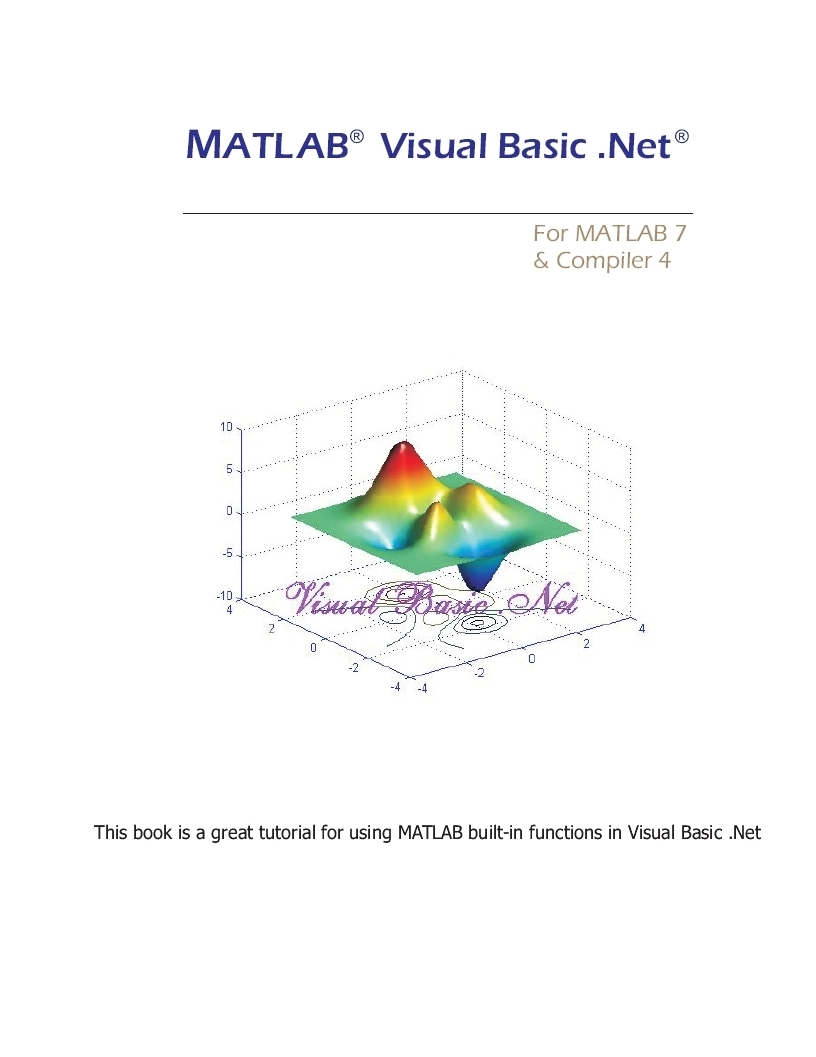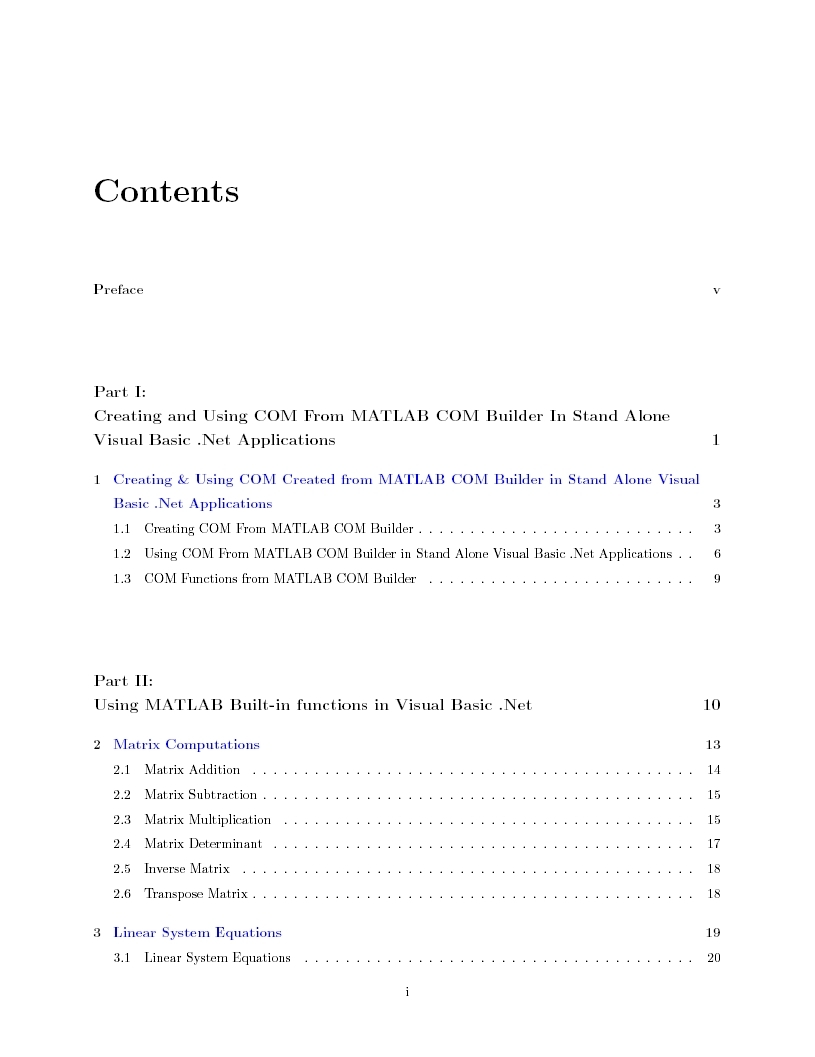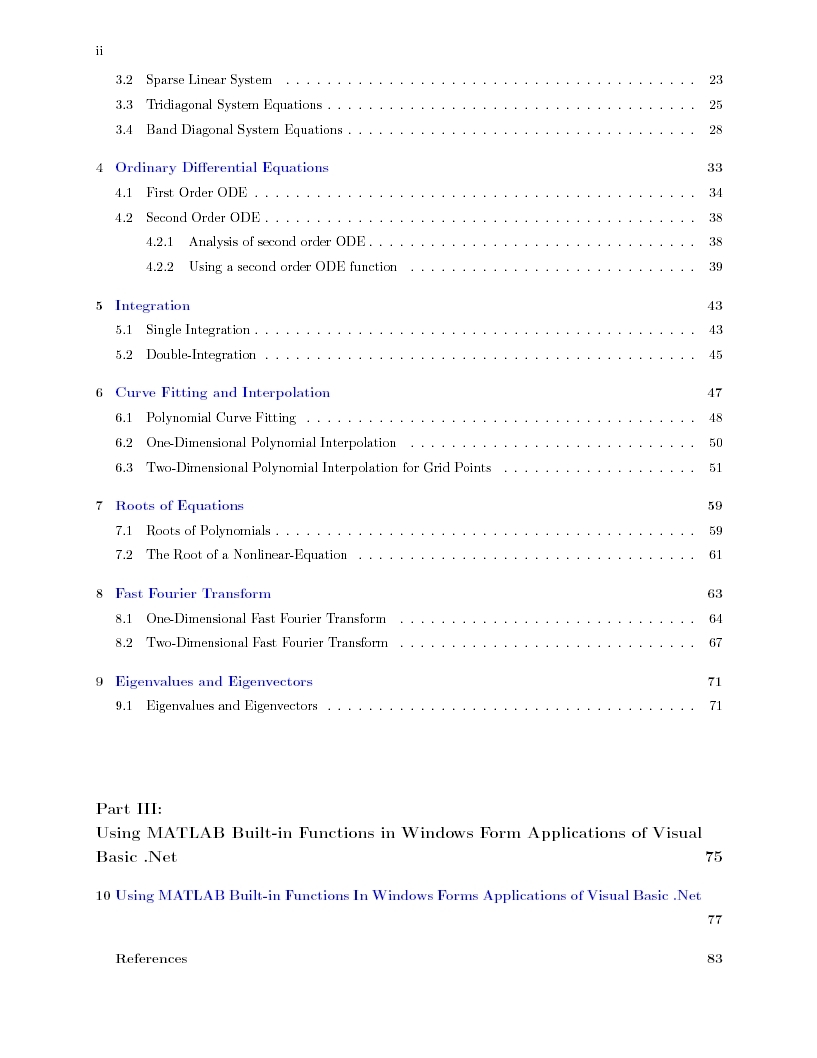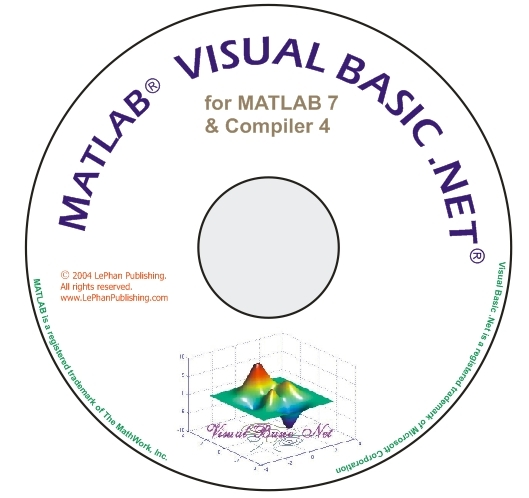MATLAB®  - Visual Basic .NET®    for Engineers

For MATLAB 2009(b) and MATLAB Compiler 4.11

MATLAB Visual Basic .NET Book illustrates the works between VB programming and MATLAB. The  book describes very detail how to use MATLAB built-in functions in VB applications. If you are a VB programmer and want to use the quality mathematical built-in functions in your VB applications, to solve mathematical problems in VB programming code, then this book is what you're looking for.

The features of this book are designed to handle following topics:

1. VB .NET functions call MATLAB built-in functions in the class created from MATLAB M-files to solve the mathematical problems.

2. VB .NET Windows Applications uses MATLAB built-in functions.

3. VB .NET functions plot figures from MATLAB Graphics.

4. VB .NET functions use API functions.

5. VB .NET functions use MATLAB Curve Fitting Toolbox functions.

6. VB .NET functions use COM generated from MATLAB M-files.

This MATLAB VB .NET Book is an ebook in the pdf format on CD-Rom which also includes the complete VB code examples of all chapters . The example codes are developed, compiled, and tested in  MATLAB 2009(b) and MATLAB Compiler 4.11 with Microsoft Visual Studio 2008, on Windows XP, Windows Vista, and Windows 7. The examples are working on scalars, vectors, and matrixes which are inputs/outputs of functions for every application. In addition, the example codes are portable and presented in the step-by-step method, therefore you can easily reuse the codes or write your own codes following the step-by-step procedure.

The MATLAB VB .NET Book is a great support for VB programmers who are using MATLAB built-in functions to develop applications and solutions. Using the combination of both tools, VB .NET and MATLAB, you have the best tool in your hand to develop and solve your  technical problems.

MATLAB Visual Basic .Net BOOK, ISBN  0-9725794-7-8

MATLAB and MATLAB Compiler are registered trademarks of The MathWork, Inc.
Microsoft, Windows, and Microsoft Visual Basic .Net are